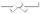# Search Results

Searching for: difference

## Individual Exercises

0 / 50

Write an `ArrayBasedStack<T>` member method called `reverseString()` that takes a String as a parameter, then uses an ArrayBasedStack...0 / 50

Write an `ArrayBasedStack<T>` member method called `decimalToBinary()` that takes a decimal as an int parameter, then uses an...0 / 10

Given a binary tree, write a recursive function to return the difference between the sum of all node values at odd levels and sum of all...0 / 10

Given a binary tree, write a recursive function to return the difference between the sum of all node values at odd levels and sum of all...